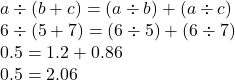## verify that a÷(b+c)#(a÷b)+(a÷c) for each of the following values of a=6,b=5 and c=7​

Question

verify that a÷(b+c)#(a÷b)+(a÷c) for each of the following values of a=6,b=5 and c=7​

in progress 0
2 months 2021-07-28T01:33:29+00:00 1 Answers 2 views 0